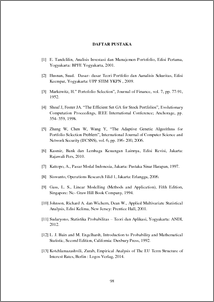# BUILDING AN OPTIMAL STOCK PORTFOLIO WITH MEAN- ABSOLUTE DEVIATION (MAD) RISK MODEL BY USING GENETIC ALGORITHM

Bintoro, Bimanto Bayu (2019) BUILDING AN OPTIMAL STOCK PORTFOLIO WITH MEAN- ABSOLUTE DEVIATION (MAD) RISK MODEL BY USING GENETIC ALGORITHM. Undergraduate thesis, UNDIP.Preview
PDF
186KbPDF
Restricted to Repository staff only

3728KbPreview
PDF
315Kb

## Abstract

In making investments, investors want the maximum level of return expectation with minimum level of risk rate. The forming of a stock portfolio is one of the ways for investors to decrease of risk rate. Return expectation and risk rate are calculate using mean absolute deviation model of portfolio theory. Then an optimal allocation of stock portfolio using genetic algorithm with real coded chromosome representation. The solution obtained from genetic algorithm are determined by the operator and the parameters used. Determination of the workings of each operator, namely crossover process, mutation, and selection. The parameters used are population size, number of generations, crossover rate, and mutation rate. Each chromosome forming stock portfolio is a candidate solution of the problem that will be evaluated based on the value of fitness. Based on the result of the forming stock portfolio using genetic algorithm can building an optimal stock of portfolio. The largest fitness obtain is 1,089176 with optimal parameters on population size is 140, number of generation is 250, crossover rate is 0,6, and mutation rate is 0,4. Keywords: genetic algorithm, mean absolute deviation model, portfolio

Item Type: Thesis (Undergraduate) Q Science > QA Mathematics Faculty of Science and Mathematics > Department of Mathematics 84234 INVALID USER 12 Jun 2022 12:29 12 Jun 2022 12:29

Repository Staff Only: item control page